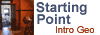# Mathematics and Statistics ModelsThis material was originally created for Starting Point:Introductory Geology
and is replicated here as part of the SERC Pedagogic Service.

## What are Mathematical and Statistical Models

These types of models are obviously related, but there are also real differences between them.

Mathematical Models:
grow out of equations that determine how a system changes from one state to the next (differential equations) and/or how one variable depends on the value or state of other variables (state equations) These can also be divided into either numerical models or analytical models.

Statistical Models:
include issues such as statistical characterization of numerical data, estimating the probabilistic future behavior of a system based on past behavior, extrapolation or interpolation of data based on some best-fit, error estimates of observations, or spectral analysis of data or model generated output.

As a way to clarify the above ideas, here is an example of the development of a simple mathematical model.

## Why use mathematical and statistical models to teach introductory courses?

Mathematical and Statistical models can be used to help students obtain a better grasp on a variety of topics.

## How can these models be used effectively in class?

In addition to the general discussion about how to use models effectively, there are a number of considerations, both pedagogical and technical, that have to do with using mathematical and statistical models specifically.
More about How to Use Mathematical and Statistical Models

## References.

Issues Related to Teaching Quantitative Skills: This page is part of the Teaching Quantitative Skills in the Geosciences website and includes a discussion of the relevance of mathematics and statistics in geoscience education.

Zachary, D. (1989). Teaching, learning, and using mathematical models in physics. Physics Education. 24(6), 339-343.

Edelstein-Keshet, K. (1988). Mathematical Models in Biology. McGraw-Hill. ISBN: 0075549506

Building the Quantitative Skills of Students in Geoscience Courses: This special issue (v48 n4, edited by Macdonald, Srogi, and Stracher) of the Journal of Geoscience Education has several examples of how mathematics and statistics can be used in geoscience courses.